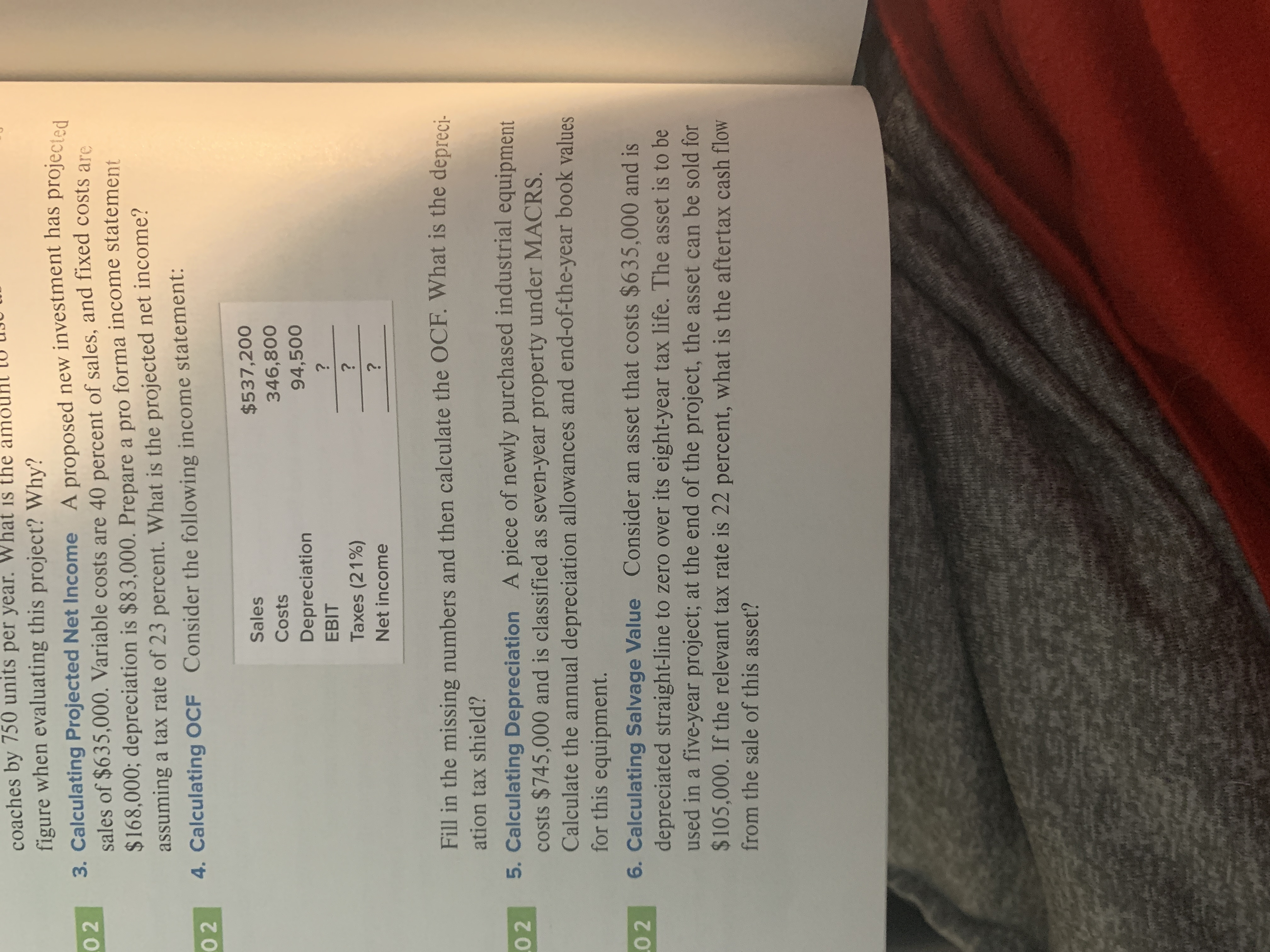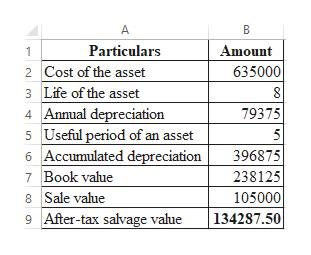# coaches by 750 units per year. Whatfigure when evaluating this project? Why?3. Calculating Projected Net Income A proposed new investment has projectedsales of \$635,000. Variable costs are 40 percent of sales, and fixed costs are\$168,000; depreciation is \$83,000. Prepare a pro forma income statementassuming a tax rate of 23 percent. What is the projected net income?is the amo0 24. Calculating OCF Consider the following income statement:02\$537,200Sales346,800Costs94,500Depreciation?EBIT?Taxes (21%)Net incomeFill in the missing numbers and then calculate the OCF. What is the depreci-ation tax shield?5. Calculating Depreciation A piece of newly purchased industrial equipmentcosts \$745,000 and is classified as seven-year property under MACRS.Calculate the annual depreciation allowances and end-of-the-year book valuesfor this equipment..0 26. Calculating Salvage Value Consider an asset that costs \$635,000 and isdepreciated straight-line to zero over its eight-year tax life. The asset is to beused in a five-year project; at the end of the project, the asset can be sold for\$105,000. If the relevant tax rate is 22 percent, what is the aftertax cash flow0 2from the sale of this asset?

Question
121 views

Number 6help_outlineImage Transcriptionclosecoaches by 750 units per year. What figure when evaluating this project? Why? 3. Calculating Projected Net Income A proposed new investment has projected sales of \$635,000. Variable costs are 40 percent of sales, and fixed costs are \$168,000; depreciation is \$83,000. Prepare a pro forma income statement assuming a tax rate of 23 percent. What is the projected net income? is the amo 0 2 4. Calculating OCF Consider the following income statement: 02 \$537,200 Sales 346,800 Costs 94,500 Depreciation ? EBIT ? Taxes (21%) Net income Fill in the missing numbers and then calculate the OCF. What is the depreci- ation tax shield? 5. Calculating Depreciation A piece of newly purchased industrial equipment costs \$745,000 and is classified as seven-year property under MACRS. Calculate the annual depreciation allowances and end-of-the-year book values for this equipment. .0 2 6. Calculating Salvage Value Consider an asset that costs \$635,000 and is depreciated straight-line to zero over its eight-year tax life. The asset is to be used in a five-year project; at the end of the project, the asset can be sold for \$105,000. If the relevant tax rate is 22 percent, what is the aftertax cash flow 0 2 from the sale of this asset? fullscreen
check_circle

Step 1

Computation of after-tax cash flow:

Hence, the after-tax cash flow is \$134,287.50.

...help_outlineImage TranscriptioncloseA Particulars Amount Cost of the asset 635000 Life of the asset 4 Annual depreciation 5 Useful period of an asset Accumulated depreciation 7 Book value 79375 5 396875 238125 8 Sale vahue 105000 134287.50 9 After-tax salvage value fullscreen

### Want to see the full answer?

See Solution

#### Want to see this answer and more?

Solutions are written by subject experts who are available 24/7. Questions are typically answered within 1 hour.*

See Solution
*Response times may vary by subject and question.
Tagged in